# Dimensioning of the metal structure Part3 (Final)

Seismic data:
See chapter on piping (paragraph 5 - 2 - e)
Class of Building: Class I
Priority Coefficient I = 1
Acceleration coefficient A = 0.08
Level of ductility ND1
Coefficient of Behavior 2
Site Coefficient S = 1
After carrying out a static analysis of the structure, the seismic loads
To complete the sizing.
Seismic loads must be applied to the framework and also the forces transmitted
To the rack by the pipe supports taken from the pipe
(See Table).

 Mounting Type Earthquake Fx (N) Earthquake Fy (N) Axial stop 116 174 Guide 1370 20
Table: Effort of the earthquake transmitted to the rack by piping

Seismic analysis:
After having dimensioned the whole structure, the seismic analysis is carried out to check whether
The chosen elements are resistant to the dynamic stresses of the earthquake by tests given by
The RPS 2000 version 2011 regulation on the ROBOT software.
Seismic calculation is a dynamic calculation or more precisely a spectral analysis. This
Analysis is divided into two parts

- A modal analysis: will allow to search the dynamic responses of a system.
- Application of the acceleration spectrum defined by RPS 2000.
The methodology of this analysis is well developed on the following flowchart:

Dimensioning the structure without taking into account the diesme => modal analysis => transforming the added mass loads => Starting the calculations => analyzing the results given by the modal analysis => the sum of% of the masses in direction XY and Z Are> 90% of the total mass => conventional seismic analysis.

One or more sum of the masses in one direction or direction is <90% mass> 70% mass seismic analysis with residual mode <70% mass seismic analysis M / sumzM ratio increase
Methodology of seismic analysis

Modal analysis:
A modal analysis characterizes the behavior of the structure from the point of view
Dynamic (soft or rigid).
The mode of modal analysis is an exact calculation method
Modes in decreasing order of the vibration periods of the most structure
As a percentage of the participating masses.
Before carrying out the modal analysis, it is necessary to transform all the load W into masses, W
Corresponds to all the permanent costs G and a fraction ψ of operating expenses
Q depending on the nature of the loads and their duration. We take :

W= G + ψ *Q
With ψ a coefficient given by the table

1 / Buildings for the use of ahbitation and administrative ... 0.20
2 / Buildings Periodically used by public 0.30
As showrooms, party room .....
3 / Use building such as restaurants, classrooms .... 0.40
4 / Building with a long operating cost 1.00
Duration such as entropot, library, silos and resevoir ...

Table : coefficient For our case ψ = 1 The calculation of the eigenmodes must be carried out in order to satisfy the following two conditions : Reach the maximum frequency of 33 Hz known as "cut-off frequency" in each Direction of excitation; Solicit 90% of the total mass M of the system in each excitation direction. 2. Calculation results - Robot -: Combinations of loads: Ultimate Limit State: 𝐸𝐿𝑈1 = 1.33 𝐺 + 1.5 𝐸𝐿𝑈2 = 1.33 + 1.42 (𝑄 + 𝑉) 𝐸𝐿𝑈3 = 𝐺 + 1.75 𝐸𝐿𝑈4 = 1.33 𝐺 + 1.5 Equation 18: Combinations in Ultimate Limit State State Limit Service: 𝐸𝐿𝑆1 = 𝐺 + 𝐸𝐿𝑆2 = 𝐺 + Equation 19: Combinations State Limit Service Limit state Accidental: 𝐴𝐶𝐶 = 𝐺 + 𝑄 + Equation 20: Combination Accidental Limit State Static calculation: Evaluation of constraints: To check the resistance of the structure, the following condition must be satisfied: ϬMax ≤ ϭe / s With: ϬMax: The maximum stress in the structure Ϭe: The yield stress of the material S: the safety coefficient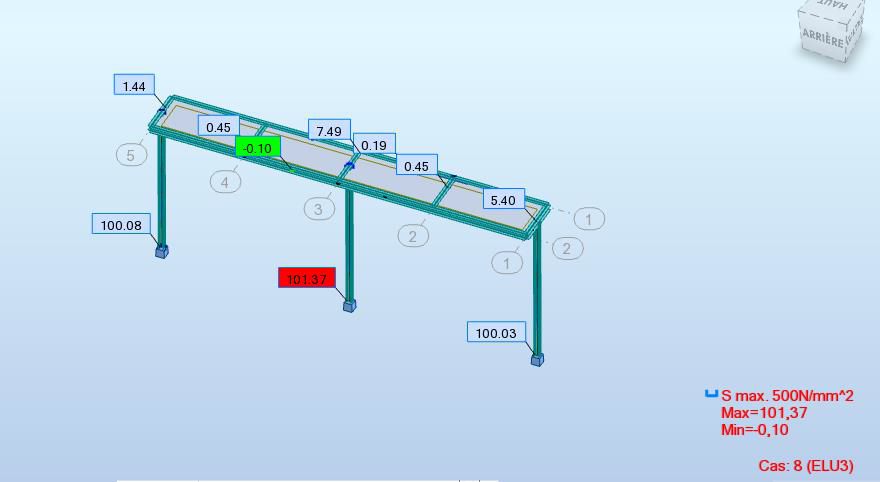Figure 28: Results of constraints

At ELU3, the most unfavorable combination corresponding to the extreme wind
ϭMax = 101,37MPa
We note that the greatest stress in the rack remains below the limit
Elasticity of S235 steel. So the resistance criterion is checked.
Evaluation of displacements:
The evaluation of displacement consists of comparing the maximum displacement in the
Structure with permissible displacement (see Figure 26)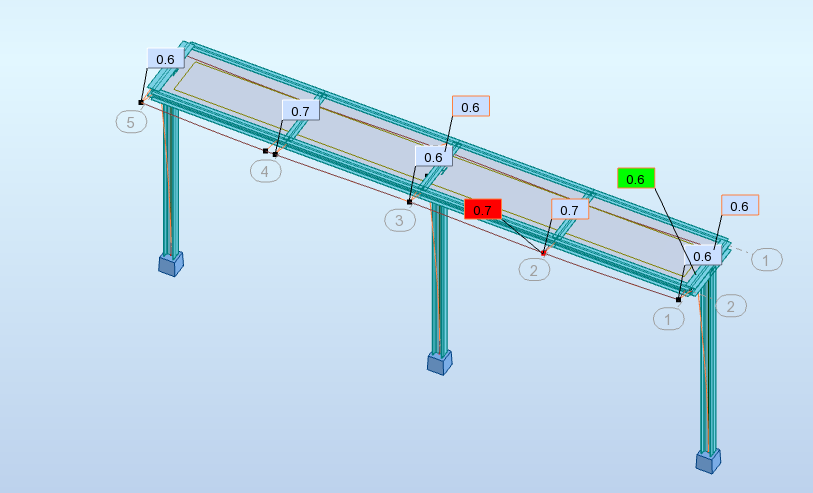Figure 29: Travel Results

At ELS1, 2 the dimensioning criterion is a maximum permissible deflection. • For vertical support elements, a head movement <L / 150 is desired. Let 0.8 <150/150 = 1 cm • For horizontal load bearing elements, an arrow at center <L / 200 is desired. 1.8 <600/200 = 3cm • Non-load-bearing elements are not checked at SLE. So the criterion is verified. Checking stability: Sleepers: The crossbars are the horizontal supporting elements, supporting the piping. Their function Main is to transmit to the poles the actions due to the piping. And in case of knots Rigid pillars, the cross-members also transmit the horizontal forces Due to wind or earthquake. They are checked only at the spill The main sleepers type 1: linked to the posts are supposed to be embedded in the They are sized for each level. Secondary sleepers type 2: linked to the longitudinal sleepers are assumed articulated The figure below illustrates the results of checking the stability of the sleepers: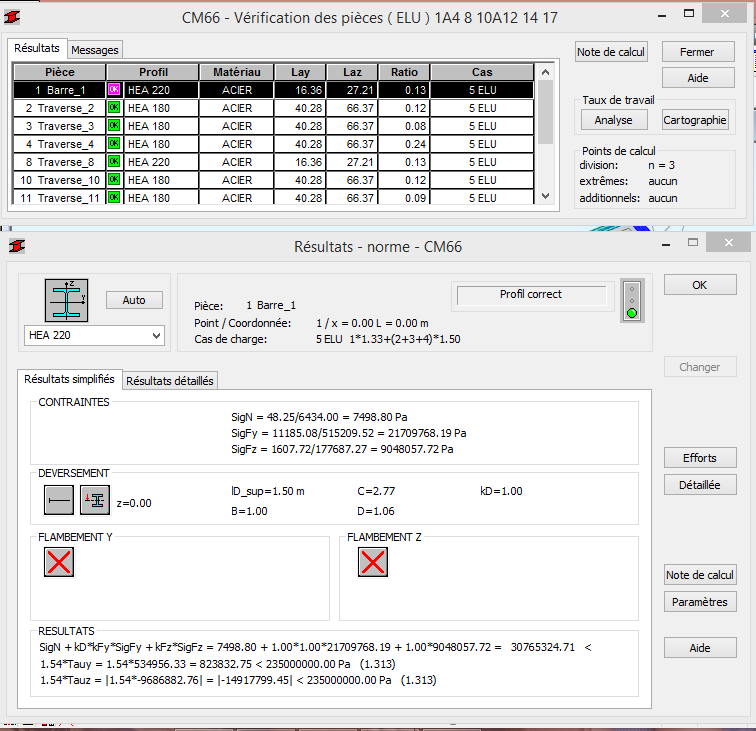Figure 30: Verification of the stability of the sleepers

It is noted that the spill is checked for the main and secondary sleepers. Posts: The columns are the vertical supporting elements of the structure. Their function is to Transfer to the foundations of the forces resulting from the actions acting through the piping and introduced By the crossbar. The posts, of length 9 m, are embedded in the plane of the planar gantry and Free in rotation along y in the global coordinate system as shown in FIG. 38. We found for section of post HEB 220. They are asked to: Bending and compression in the plane of the gantries. Simple compression in the long plane of the rack. Transverse actions due to wind.
They are verified by buckling, following the two planes (y, z) of the local reference of the column, the
Length of the buckling is calculated taking into account the assembled transverse beams
With the post. (Figure 28).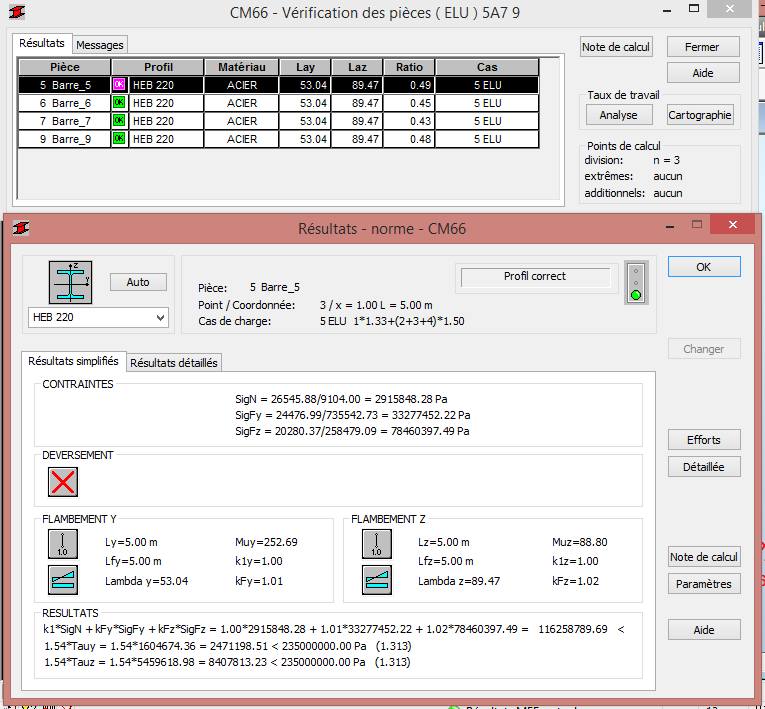Figure 31: Checking the stability of the posts

It is noted that the posts are also checked at buckling.
Dynamic calculation:
Results of the modal calculation:
Table 38 represents the totality of eigenmodes with frequency, period and masses
cumulated. We note that the cumulative mass reaches 90% for both directions
X and Y from mode 3 without reaching the cut-off frequency (see Table 35).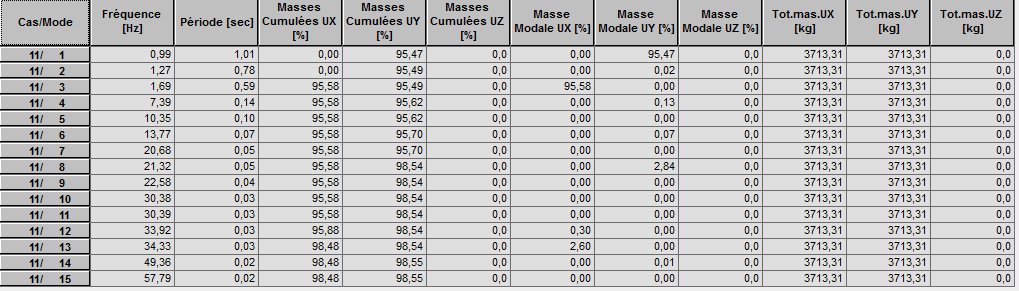Table 35: Modal Analysis Results

Results of the Seismic Analysis: The dynamic approach is based on: The maximum response of the structure by means of site-appropriate response spectra construction A direct calculation as a function of time by the use of accelerograms adapted to the Site of construction. We investigated the maximum effects of the earthquake, using the ROBOT software, on Our structure. The fundamental combination of the actions to be taken into account for the calculation of The verification of the limit states is given by the following expression: 𝑺𝒄 = 𝑮 + 𝑺 + With: G: Dead weight and long-term permanent loads S: Effects of the earthquake Q: Operating Expenses Ψ = 1, accompanying factor whose values ​​are given in table 40 Verification of displacement: The aim is to verify if the displacement of the structure does not exceed the acceptable limits Even taking into account the effects of the earthquake. It should be verified that under the effect of the overall actions the deformations of the elements Of the structure remain limited to the maximum values ​​set by the RPS Regulation 2000. (Figure 29).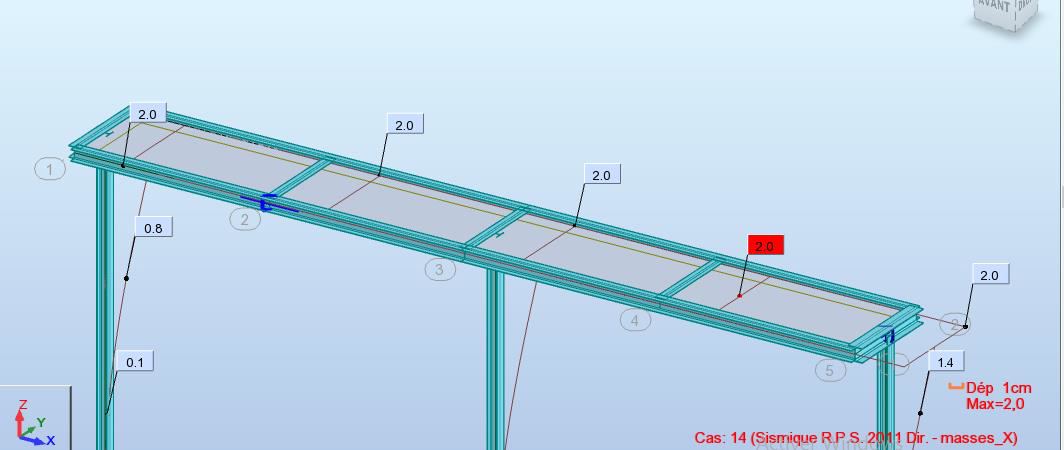Figure 32: Extreme movements (earthquake)

The verification is carried out according to the RPS2000 standard, which requires the verification of the displacements
following :
The inter-stage lateral displacements Δel evaluated from the calculation actions must
Be limited to:
K Δel ≤ 0.007 h for Class I buildings
H: being the height of the stage
K = 3: coefficient of behavior
Either: 3 * 2≤0,007 * 950 6≤6,65
Therefore, the interstage lateral displacements are checked
The total lateral displacement of the rack Δg must be limited to:
Δg ≤ 0.004 H
Let 2 <0.004 * 950 2≤3.8
H: being the total height of the structure
e. Study of assemblies:
In this part, we will check the assemblies on the ROBOT software. We will study
Two assembly cases for bar 6 at node 17, since it is the most stressed bar, and
The embedded post foot (Figure 30)Figure 33: Diagram of the beam-post assembly and the embedded pole foot
Pole Beam Assembly:

### Dimensioning of the metal structure Part3 (Final)

Seismic data:
See chapter on piping (paragraph 5 - 2 - e)
Class of Building: Class I
Priority Coefficient I = 1
Acceleration coefficient A = 0.08
Level of ductility ND1
Coefficient of Behavior 2
Site Coefficient S = 1
After carrying out a static analysis of the structure, the seismic loads
To complete the sizing.
Seismic loads must be applied to the framework and also the forces transmitted
To the rack by the pipe supports taken from the pipe
(See Table).

 Mounting Type Earthquake Fx (N) Earthquake Fy (N) Axial stop 116 174 Guide 1370 20
Table: Effort of the earthquake transmitted to the rack by piping

Seismic analysis:
After having dimensioned the whole structure, the seismic analysis is carried out to check whether
The chosen elements are resistant to the dynamic stresses of the earthquake by tests given by
The RPS 2000 version 2011 regulation on the ROBOT software.
Seismic calculation is a dynamic calculation or more precisely a spectral analysis. This
Analysis is divided into two parts

- A modal analysis: will allow to search the dynamic responses of a system.
- Application of the acceleration spectrum defined by RPS 2000.
The methodology of this analysis is well developed on the following flowchart:

Dimensioning the structure without taking into account the diesme => modal analysis => transforming the added mass loads => Starting the calculations => analyzing the results given by the modal analysis => the sum of% of the masses in direction XY and Z Are> 90% of the total mass => conventional seismic analysis.

One or more sum of the masses in one direction or direction is <90% mass> 70% mass seismic analysis with residual mode <70% mass seismic analysis M / sumzM ratio increase
Methodology of seismic analysis

Modal analysis:
A modal analysis characterizes the behavior of the structure from the point of view
Dynamic (soft or rigid).
The mode of modal analysis is an exact calculation method
Modes in decreasing order of the vibration periods of the most structure
As a percentage of the participating masses.
Before carrying out the modal analysis, it is necessary to transform all the load W into masses, W
Corresponds to all the permanent costs G and a fraction ψ of operating expenses
Q depending on the nature of the loads and their duration. We take :

W= G + ψ *Q
With ψ a coefficient given by the table

1 / Buildings for the use of ahbitation and administrative ... 0.20
2 / Buildings Periodically used by public 0.30
As showrooms, party room .....
3 / Use building such as restaurants, classrooms .... 0.40
4 / Building with a long operating cost 1.00
Duration such as entropot, library, silos and resevoir ...

Table : coefficient For our case ψ = 1 The calculation of the eigenmodes must be carried out in order to satisfy the following two conditions : Reach the maximum frequency of 33 Hz known as "cut-off frequency" in each Direction of excitation; Solicit 90% of the total mass M of the system in each excitation direction. 2. Calculation results - Robot -: Combinations of loads: Ultimate Limit State: 𝐸𝐿𝑈1 = 1.33 𝐺 + 1.5 𝐸𝐿𝑈2 = 1.33 + 1.42 (𝑄 + 𝑉) 𝐸𝐿𝑈3 = 𝐺 + 1.75 𝐸𝐿𝑈4 = 1.33 𝐺 + 1.5 Equation 18: Combinations in Ultimate Limit State State Limit Service: 𝐸𝐿𝑆1 = 𝐺 + 𝐸𝐿𝑆2 = 𝐺 + Equation 19: Combinations State Limit Service Limit state Accidental: 𝐴𝐶𝐶 = 𝐺 + 𝑄 + Equation 20: Combination Accidental Limit State Static calculation: Evaluation of constraints: To check the resistance of the structure, the following condition must be satisfied: ϬMax ≤ ϭe / s With: ϬMax: The maximum stress in the structure Ϭe: The yield stress of the material S: the safety coefficientFigure 28: Results of constraints

At ELU3, the most unfavorable combination corresponding to the extreme wind
ϭMax = 101,37MPa
We note that the greatest stress in the rack remains below the limit
Elasticity of S235 steel. So the resistance criterion is checked.
Evaluation of displacements:
The evaluation of displacement consists of comparing the maximum displacement in the
Structure with permissible displacement (see Figure 26)Figure 29: Travel Results

At ELS1, 2 the dimensioning criterion is a maximum permissible deflection. • For vertical support elements, a head movement <L / 150 is desired. Let 0.8 <150/150 = 1 cm • For horizontal load bearing elements, an arrow at center <L / 200 is desired. 1.8 <600/200 = 3cm • Non-load-bearing elements are not checked at SLE. So the criterion is verified. Checking stability: Sleepers: The crossbars are the horizontal supporting elements, supporting the piping. Their function Main is to transmit to the poles the actions due to the piping. And in case of knots Rigid pillars, the cross-members also transmit the horizontal forces Due to wind or earthquake. They are checked only at the spill The main sleepers type 1: linked to the posts are supposed to be embedded in the They are sized for each level. Secondary sleepers type 2: linked to the longitudinal sleepers are assumed articulated The figure below illustrates the results of checking the stability of the sleepers:Figure 30: Verification of the stability of the sleepers

It is noted that the spill is checked for the main and secondary sleepers. Posts: The columns are the vertical supporting elements of the structure. Their function is to Transfer to the foundations of the forces resulting from the actions acting through the piping and introduced By the crossbar. The posts, of length 9 m, are embedded in the plane of the planar gantry and Free in rotation along y in the global coordinate system as shown in FIG. 38. We found for section of post HEB 220. They are asked to: Bending and compression in the plane of the gantries. Simple compression in the long plane of the rack. Transverse actions due to wind.
They are verified by buckling, following the two planes (y, z) of the local reference of the column, the
Length of the buckling is calculated taking into account the assembled transverse beams
With the post. (Figure 28).Figure 31: Checking the stability of the posts

It is noted that the posts are also checked at buckling.
Dynamic calculation:
Results of the modal calculation:
Table 38 represents the totality of eigenmodes with frequency, period and masses
cumulated. We note that the cumulative mass reaches 90% for both directions
X and Y from mode 3 without reaching the cut-off frequency (see Table 35).Table 35: Modal Analysis Results

Results of the Seismic Analysis: The dynamic approach is based on: The maximum response of the structure by means of site-appropriate response spectra construction A direct calculation as a function of time by the use of accelerograms adapted to the Site of construction. We investigated the maximum effects of the earthquake, using the ROBOT software, on Our structure. The fundamental combination of the actions to be taken into account for the calculation of The verification of the limit states is given by the following expression: 𝑺𝒄 = 𝑮 + 𝑺 + With: G: Dead weight and long-term permanent loads S: Effects of the earthquake Q: Operating Expenses Ψ = 1, accompanying factor whose values ​​are given in table 40 Verification of displacement: The aim is to verify if the displacement of the structure does not exceed the acceptable limits Even taking into account the effects of the earthquake. It should be verified that under the effect of the overall actions the deformations of the elements Of the structure remain limited to the maximum values ​​set by the RPS Regulation 2000. (Figure 29).Figure 32: Extreme movements (earthquake)

The verification is carried out according to the RPS2000 standard, which requires the verification of the displacements
following :
The inter-stage lateral displacements Δel evaluated from the calculation actions must
Be limited to:
K Δel ≤ 0.007 h for Class I buildings
H: being the height of the stage
K = 3: coefficient of behavior
Either: 3 * 2≤0,007 * 950 6≤6,65
Therefore, the interstage lateral displacements are checked
The total lateral displacement of the rack Δg must be limited to:
Δg ≤ 0.004 H
Let 2 <0.004 * 950 2≤3.8
H: being the total height of the structure
e. Study of assemblies:
In this part, we will check the assemblies on the ROBOT software. We will study
Two assembly cases for bar 6 at node 17, since it is the most stressed bar, and
The embedded post foot (Figure 30)Figure 33: Diagram of the beam-post assembly and the embedded pole foot
Pole Beam Assembly: